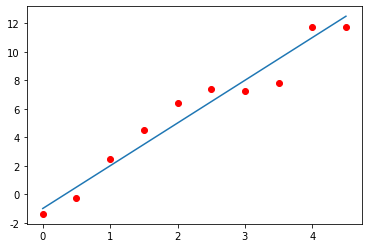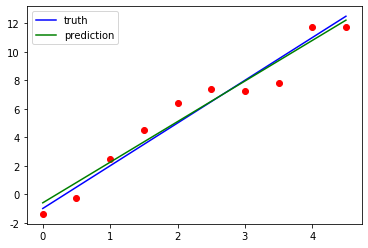import numpy as np
import matplotlib.pyplot as plt

%run import_npnet.py
npnet = import_npnet(1)


# 模型

# import
class Model:

def __init__(self, **kwargs):
self.kwargs = kwargs
self.parameters = {}

@property
def _parameters(self):
if isinstance(self.parameters, list):
return self.parameters
return [self.parameters]

@property

for name in params:

def forward(self, input):
raise NotImplementedError()

raise NotImplementedError()

def __call__(self, *args, **kwargs):
return self.forward(*args, **kwargs)

def __repr__(self):
return f"<{self.__class__.__name__}>"


Model 中定义了两个属性 (property) Model._parametersModel._gradients。这两个属性返回对应属性的列表 (list) 形式。比如 Model._parameters 检查 Model.parameters 是否为列表，若是则直接返回，若不是则返回只有其一个元素的列表。这两个属性是为了方便以后的实现。我们还定义了 Model._init_gradients。当我们定义好 Model.parameters 之后，我们可以用这个方法初始化所有偏导数为0。

## 线性回归

$$y = x W + b,$$

$$y_{ij} = \sum_{k=1}^m x_{ik} W_{kj} + b_j.$$

$$\frac{\partial L}{\partial b_h} = \sum_{i, j} \frac{\partial L}{\partial y_{ij}} \cdot \frac{\partial y_{ij}}{\partial b_h} = \sum_{ij} g_{i,j}\delta_{jh} = \sum_{i=1}^l g_{ih}.$$

$$\frac{\partial L}{\partial W_{uv}} = \sum_{i, j} \frac{\partial L}{\partial y_{ij}} \cdot \frac{\partial y_{ij}}{W_{uv}} = \sum_{i, j} g_{ij} x_{iu} \delta_{jv} = \sum_{i=1}^l g_{iv}x_{iu}.$$

$$\frac{\partial L}{\partial x_{uv}} = \sum_{i, j} \frac{\partial L}{\partial y_{ij}} \cdot \frac{\partial y_{ij}}{\partial x_{uv}} = \sum_{i, j} g_{ij}W_{vj}\delta_{iu} = \sum_{j=1}^n g_{uj}W_{vj}.$$

# import
class Linear(Model):

def __init__(self, in_features, out_features):
super().__init__(in_features=in_features,
out_features=out_features)
m, n = in_features, out_features
params = self.parameters

params['W'] = np.random.randn(m, n)
params['W'] *= np.sqrt(2.0 / (m + n))
params['b'] = np.zeros(n)

def forward(self, input):
params = self.parameters
self.input = input
output = input @ params['W'] + params['b']
return output



# 标准

# import
class Criterion:

def __init__(self, *args, **kwargs):
pass

def forward(self, output, target):
raise NotImplementedError()

raise NotImplementedError()

def __call__(self, *args, **kwargs):
return self.forward(*args, **kwargs)

def __repr__(self):
return f"<{self.__class__.__name__}>"


## 均方误差

$$L(y, \tilde{y}) = \frac{1}{2} |y - \tilde{y}|_2^2.$$

$$|x|_2^2 = \sum_{i=1}^n x_i^2.$$

$$\frac{\partial L}{\partial y} = y - \tilde{y}.$$
# import
class MSELoss(Criterion):

def forward(self, output, target):
self.output = output
self.target = target
n = output.shape
loss = np.sum((output - target) ** 2)
loss /= (2 * n)
self.loss = loss
return loss

n = self.output.shape
return (self.output - self.target) * grad / n


# 优化器

# import
class Optimizer:

def __init__(self, model, lr=1e-3, *args, **kwargs):
self.model = model
self.parameters = model._parameters
self.lr = lr

def step(self):
raise NotImplementedError()

g.fill(0.0)

def __repr__(self):
return f"<{self.__class__.__name__}>"


## 随机梯度下降

# import
class SGD(Optimizer):

def step(self):
lr = self.lr
for name in params.keys():


# 导数验证

$$\frac{f(x+h) - f(x-h)}{2h}.$$

# import

def __init__(self, model, input=None, input_shape=None,
sample=100, h=1e-4, allowed_error=1e-7):
self.model = model
self.input = input
if input is None:
self.input = np.random.randn(*input_shape)
self.sample = sample
self.h = h
self.allowed_error = allowed_error

self.criterion = MSELoss()
output = self.forward(self.input)
self.target = np.zeros_like(output)
self.criterion(output, self.target)

def check(self):
return False
parameters = self.model._parameters
for name in params.keys():
return False
return True

shape = comp.shape
input = self.input
h, sample = self.h, self.sample
criterion, model = self.criterion, self.model
target, allowed_error = self.target, self.allowed_error
for _ in range(sample):
point = tuple(np.random.randint(n) for n in shape)
comp[point] += h
high = criterion(self.forward(input), target)
comp[point] -= 2 * h
low = criterion(self.forward(input), target)
comp[point] += h
numerical_grad_at_point = (high - low) / (2 * h)
if error > allowed_error:
return False
return True

def forward(self, *args, **kwargs):
return self.model.forward(*args, **kwargs)

linear = Linear(10, 20)

True

# 直线拟合

x = np.arange(0, 5, 0.5).reshape(-1, 1)
z = 3 * x - 1
y = z + np.random.randn(len(x)).reshape(-1, 1)
plt.scatter(x, y, c='r')
plt.plot(x, z)
plt.show()#建立模型
model = Linear(1, 1)

# 记录下当前模型的初始值供后面的使用
a = model.parameters['W']
b = model.parameters['b']

# 选定超参数 (hyperparameter)
lr = 0.1

# 选择标准以及优化器
criterion = MSELoss()
optim = SGD(model, lr=lr)

# 训练模型
for _ in range(100):
output = model(x) # 预测
loss = criterion(output=output, target=y) # 计算损失
optim.step() # 使用优化器更新参数

# 检测模型
plt.scatter(x, y, c='r')
plt.plot(x, z, 'b', label='truth')
plt.plot(x, model(x), 'g', label='prediction')
plt.legend()
plt.show()n = len(x)
sx, sy, sxy, sx2 = np.sum(x), np.sum(y), np.sum(x * y), np.sum(x ** 2)
for _ in range(100):
ga = (sx2 * a + sx * b - sxy) / n
gb = (sx * a - sy) / n + b
a -= lr * ga
b -= lr * gb

print(f'a: {a:.8f}, b: {b:.8f}')
print(model.parameters)

a: 2.85006934, b: -0.60110195
{'W': array([[2.85006934]]), 'b': array([-0.60110195])}